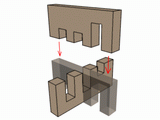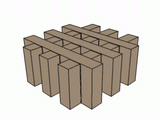### Problem Statement

The goal of Notched Wood Bars puzzle is to complete the square lattice by inserting the given bars into each other. Each element of the puzzle is a rectangular bar with two or more notches. Each notch has only two kinds of depth, either shallow or deep.

The lattice has to be constructed by inserting the bars vertically from below and horizontally from above or vice versa, matching each shallow notch with a deep one. Each bar can therefore be oriented with the notches facing upwards or downwards.

``````

You have 2*K bars in the puzzle, each with K notches, and you must determine the number of ways the lattice can be constructed. Notice that you must count only distinct configurations. Two configurations are considered distinct if one is not a rotation of the other. More specifically, if one configuration can be rotated in any way (in all three dimensions) such that all of its pieces exactly coincide with identical pieces in another configuration, then the two configurations are not distinct.

You will be given a String[] bars. Each element of bars represents the notches on a single bar. 'S' represents a shallow notch, and 'D' represents a deep notch. Return the number of distinct ways to complete the puzzle, or 0 if the puzzle is incorrect and cannot be completed.

### Definition

 Class: NotchedWoodBarsPuzzle Method: countSolutions Parameters: String[] Returns: int Method signature: int countSolutions(String[] bars) (be sure your method is public)

### Constraints

-bars will contain exactly 4, 6, or 8 elements.
-The number of characters in each element of bars will be equal to the number of elements in bars divided by 2.
-Each element in bars will consist of only 'S' and 'D' characters.

### Examples

0)

 `{"SD", "SD", "SD", "SD"}`
`Returns: 1`
 The only way to solve this puzzle is to insert "SD" and "DS" from below, and "DS" and "SD" from above. All other correct configurations are rotations of this one.
1)

 `{"SDS", "SDS", "SDS", "DDD","SSS","DDD"}`
`Returns: 2`
 There are two ways to solve this puzzle. We can insert from below either "SDS", "SDS" and "SDS" or "SDS", "SSS" and "SDS".
2)

 `{"SDD", "SSS", "SDS", "DDD", "SDD", "DSS"}`
`Returns: 9`
3)

 `{"SDDS", "SDDS", "SDDS", "SDDS", "SDDS", "SDDS", "SDDS", "SDDS"}`
`Returns: 0`
4)

 `{"DSDD", "DDSD", "SDDD", "SSSS", "DSSD", "DSSS", "SSDD", "SDSD"}`
`Returns: 80`
5)

 `{"SSSS","SSSS","SSSS","SSSS","DDDD","DDDD","DDDD","DDDD"}`
`Returns: 1`

#### Problem url:

http://www.topcoder.com/stat?c=problem_statement&pm=6073

#### Problem stats url:

http://www.topcoder.com/tc?module=ProblemDetail&rd=9984&pm=6073

Andrew_Lazarev

#### Testers:

PabloGilberto , lbackstrom , brett1479 , radeye , Olexiy

Brute Force